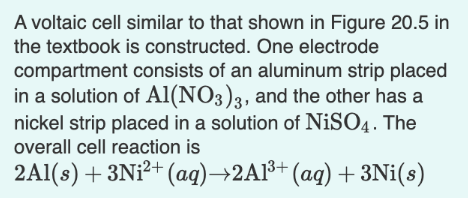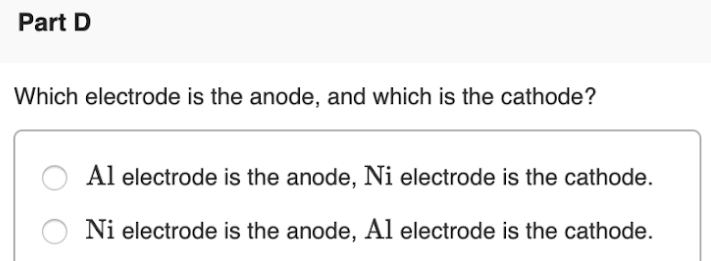# A voltaic cell similar to that shown in Figure 20.5 in the textbook is constructed. One electrode compartment consists of an aluminum strip placed in a solution of Al(NO3)3, and the other has a nickel strip placed in a solution of NiSO4. The overall call reaction is 2Al(s) + 3Ni^2+(aq) → 2Al^3=(aq) + 3Ni(s) Which electrode is the anode, and which is the cathode?